# Basic Arithmetic : Multiplication and Division with Money

## Example Questions

### Example Question #1 : Multiplication And Division With Money

Leon boughtpairs of socks that cost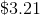per pair,shirts that cost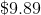each, and a dozen pencils that cost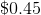for. How much did Leon spend in total?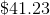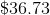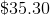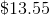Explanation:

First, let's find the cost of the socks Leon bought.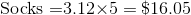Then find the total cost of the shirts Leon bought.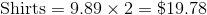Next, find the cost of the pencils. Watch out here because the pencils are priced per 6. There are two 6's in a dozen.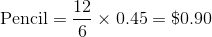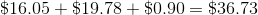### Example Question #1 : Multiplication And Division With Money

Molly boughtposters and paid a total of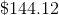. What was the cost of each poster?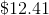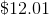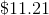Explanation:

To find the cost of each individual poster, divide the total cost by the number of posters Molly bought.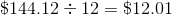### Example Question #1 : Multiplication And Division With Money

If John has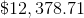in his savings account, and he accrues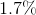% interest on that savings account every year, how much money will John have in his account next year? All answers have been rounded to the nearest cent.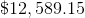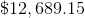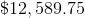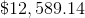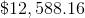Explanation:

For this problem, first you have to calculate the interest, and then add it to the toal in the bank account.

To calculate interest, convert the percent into a decimal 1.7% = .017, and multiply it by the total amount.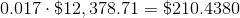is your interest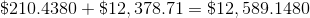is your total

round to the nearest cent and you arrive at.

### Example Question #1 : Multiplication And Division With Money

Anne worked at a coffee shop, where she made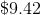an hour. She left for a job as a receptionist, where she makes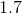times more money per hour. How much money will she make working an eight hour day at her new job? The answer has been rounded to the nearest whole cent.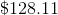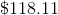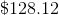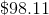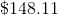Explanation:

To do this problem, first break it into smaller parts.  First, let's find Anne's wage as a receptionist: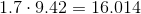Then, take her new hourly wage,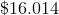and multiply it by eight, for eight hours worked: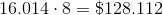Finally, round to the nearest whole cent, and you get.

### Example Question #42 : Money And Time

Francis has to pay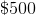per month in rent, plus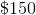for heat and electricity, and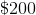for food.  Francis worksdays a month, and he earns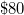per day.  How much money does Francis have left over at the end of the month?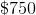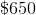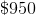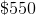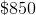Explanation:

Break the problem into two parts.  First, add up Francis' expenses: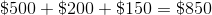Then, calculate Francis' income: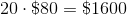Finally, subtract his expenses from his income: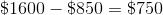### Example Question #1 : Multiplication And Division With Money

Susan works 20 hours per week at a rate of \$17/hour. How much money (in dollars) will Susan make in a month?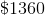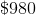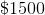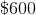Explanation: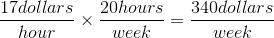There are 4 weeks in a month, so: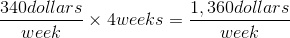### All Basic Arithmetic Resources